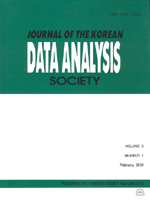상세검색
최근 검색어 전체 삭제
다국어입력
즐겨찾기0KCI등재 학술저널

# Fitting Proportional Odds Survival Models with Random Effects via Binominal HGLMs

• 등재여부 : KCI등재
• 2010.12
• 2953 - 2962 (10 pages)

Recently, survival models with random effects have been widely used for the analysis of correlated survival data. In particular, frailty models(FMs) and proportional odds models (POMs) with random effects are useful. The FMs are based on the assumption of proportional hazards(PH), whereas POMs are not. The POM is a flexible non-PH model and give an interpretation of odds ratio in survival analysis. However, it is usually difficult to fit POMs with random effects because of integration about random effects and censoring. The hierarchical-likelihood for the random-effect POMs can be expressed as that for binominal hierarchical generalized linear models(HGLMs). Thus, in this paper we show how to fit easily the POMs via HGLMs. The proposed method is illustrated using a well-known correlated survival data set.

1. Introduction

2. A classical proportional odds model

3. Fitting proportional odds models with random effects

4. Illustration

5. Discussion

References